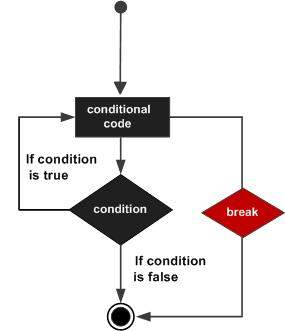R - Break Statement

The break statement in R programming language has the following two usages −

• When the break statement is encountered inside a loop, the loop is immediately terminated and program control resumes at the next statement following the loop.

• It can be used to terminate a case in the switch statement (covered in the next chapter).

Syntax

The basic syntax for creating a break statement in R is −

break

Flow DiagramExample

v <- c("Hello","loop")
cnt <- 2

repeat {
print(v)
cnt <- cnt + 1

if(cnt > 5) {
break
}
}

When the above code is compiled and executed, it produces the following result −

 "Hello" "loop"
 "Hello" "loop"
 "Hello" "loop"
 "Hello" "loop"
r_loops.htm